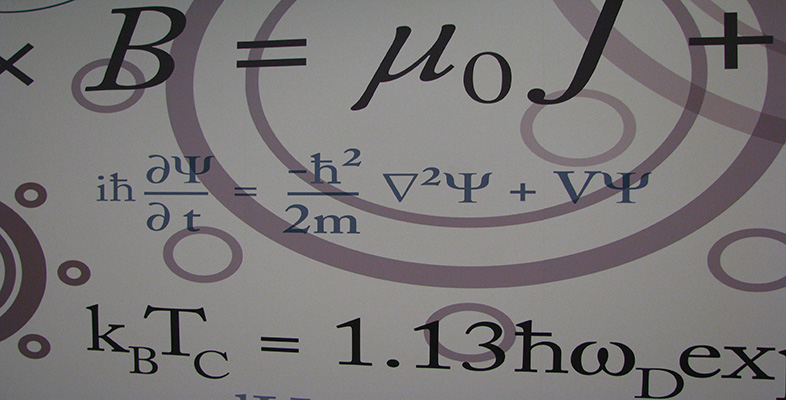Number systems

This free course is available to start right now. Review the full course description and key learning outcomes and create an account and enrol if you want a free statement of participation.

Free course

2.2 The complex plane

Just as there is a one-one correspondence between the real numbers and the points on the real line, so there is a one-one correspondence between the complex numbers and the points in the plane. This correspondence is given by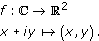Thus we can represent points in the plane by complex numbers and, conversely, we can represent complex numbers by points in the plane. When we represent complex numbers by points in the plane, we refer to the plane as the complex plane, and we often refer to the complex numbers as points in the complex plane. A diagram showing complex numbers represented as points in the plane in this way is sometimes called an Argand diagram.

The French mathematician Jean-Robert Argand's publication of the idea in 1806 was the first to be generally recognised.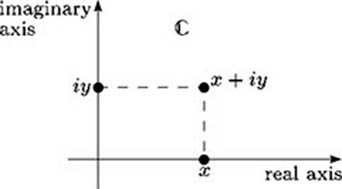Real numbers are represented in the complex plane by points on the x-axis; this axis is called the real axis. Similarly, numbers of the form iy are represented by points on the y-axis; this axis is called the imaginary axis.

Exercise 7

Draw a diagram showing each of the following points in the complex plane: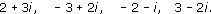Solution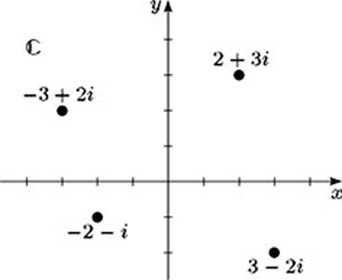M208_6